# Vector – Mathematics

## Introduction

A vector is a mathematical tool that allows us to represent magnitudes in which not only the intensity (or modulus), but also the direction and direction in which they are applied are important.

The vectors are very useful in the development of games, they allow us to define directions for movement, do ray tracing, among other applications.

The simplest and perhaps the most everyday example I can think of is that of a force. Forces are vector magnitudes, not only does the amount of force applied matter, but also direction and meaning.

If, for example, we change the direction of the force we apply to an object, the object will probably move in the opposite direction.

Other examples of vector magnitudes can be torque, angular momentum, velocity. In electricity and magenithm we have the electric field at one point, the Poynting Vector.

# I MADE THIS GAME IN 72 HOURS

CONSIDER SUBSCRIBING!

# LET'S CONTINUE WITH THE ARTICLE

## Vectors in the plane and in space

For practical reasons we are going to look at the case of vectors in the plane and in space, i.e. vectors of two and three components respectively.

### Mathematically

Usually a vector is identified by a letter with an arrow at the top.

There are different types of notations, one of them is to write the components of the vector in parentheses and separated with comma. In the plane we use two components and in the space three.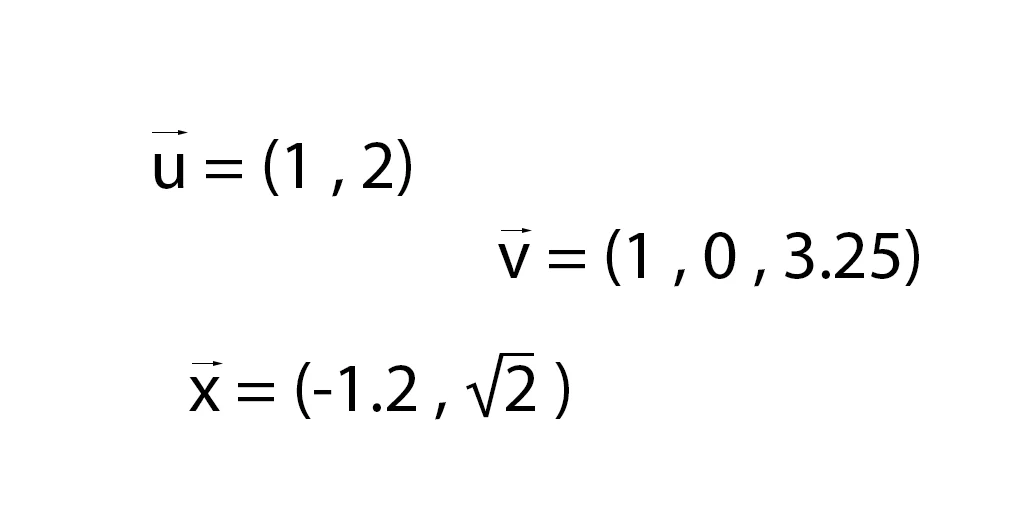Fig. 1: Examples of vectors in the plane and in space.

### Graphic Representation

The value of the vector represents the final point of an arrow starting from the origin of coordinates (i.e. (0.0) in the plane and (0.0.0) in space), to the coordinate indicated by the vector. As illustrated in figure 2.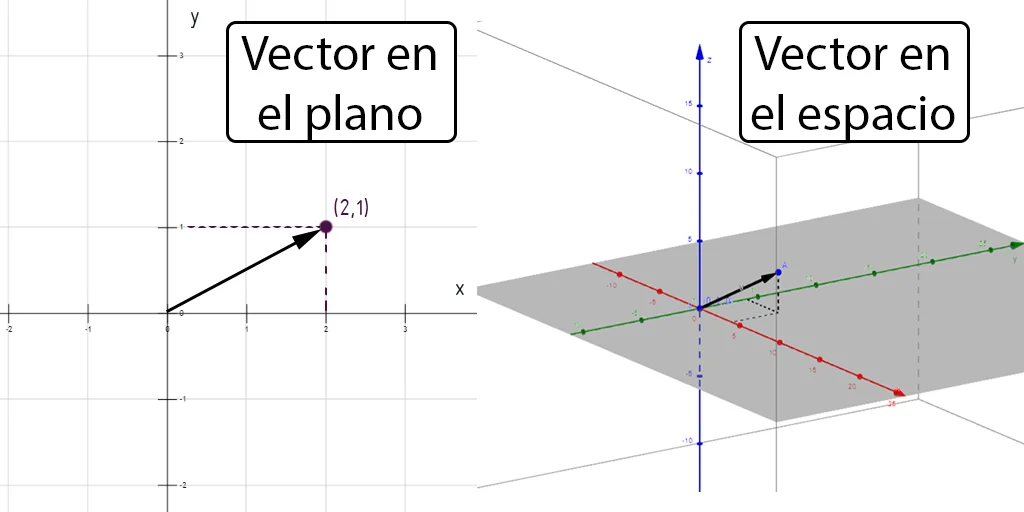Fig. 2: Graphic representation of vectors in the plane (left) and vectors in space (right).

## Characteristics of a vector

### Components

The components are the actual values for each axis of the coordinate system.

In the plane a vector has two components, generally x and y, in space we need three components, in general they are called x, y and z.

### Magnitude

The module, norm or magnitude of a vector tells us about the size of the vector, the magnitude or intensity it has, in other words is how much the vector measures from the origin to the final point.

To calculate the module, Pitagoras’ theorem is used on the right triangles formed by the vector with the coordinate axes. This sounds somewhat complicated but the formulas are simple, in figure 3 we see the calculation of the norm of a vector in the plane and in space. Formula with examples.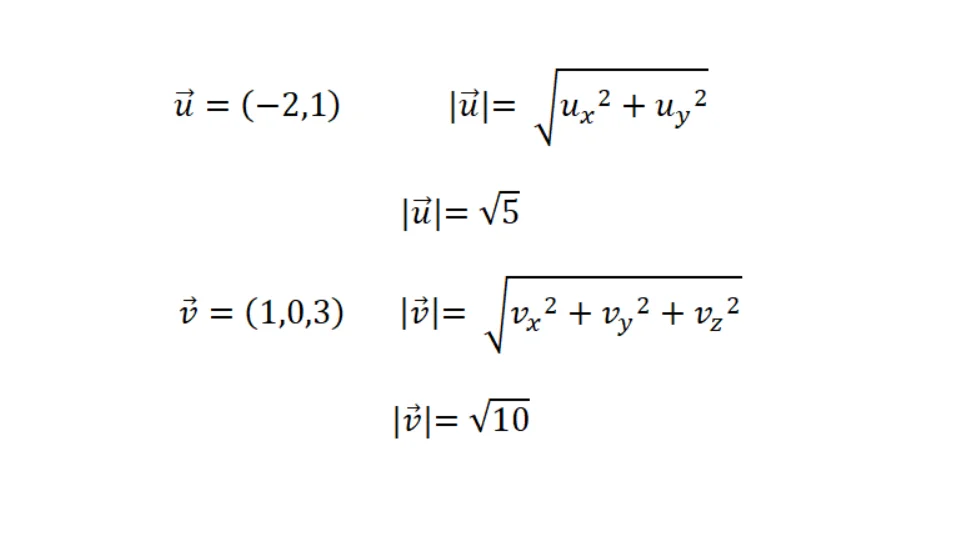Fig. 3: Calculation of the module or norm of a vector in the plane and in space.

### Orientation

The orientation of the vector in the plane or in space. This allows us to calculate angles with respect to coordinate axes using right triangles, sinuses and cosines.

Given a vector we can find a single line that contains it.

Here we begin to see the usefulness of knowing about vectors to program video games, with the vectors we can represent movements in a determined direction or calculate the trajectory of a projectile for example.

### Sense

Given a direction for the vector, we said that there is only one line that contains it, however the vector could be pointing to one side or the other of the line. With meaning we solve this ambiguity.

To change the direction of a vector it is enough to multiply by -1 all its components.

## Vector in mathematics vs Vector in programming

In programming there is also a type of data known as vectors, arrays or arrays. However, this refers to a programming structure in which we can order data of the same type and use them to solve algorithms.

We can model mathematical vectors using arrays, however arrays can contain strings of text for example.

## Mathematical Vectors in Unity

In Unity there are several components that use vectors, the simplest of which is the Transform component that determines the position, rotation and scale of a GameObject in Unity.

For this it uses three three-dimensional vectors. In figure 4 we can see these vectors in the inspector. Unity gives the vector components the name x, y and z.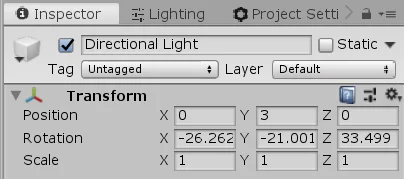Fig. 4: Transform component that has all GameObject in Unity.

Other components such as Colliders use vectors to draw the Collider, define its position and size. In figure 5 we see an example of Collider in three dimensions in which three component vectors are used and in figure 6 an example of Collider 2D in which two component vectors are used.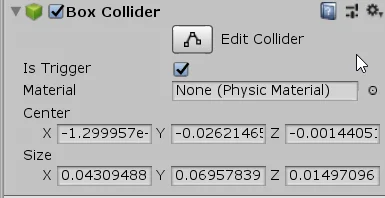Fig. 5: 3-component vectors in for the diensions of a Collider.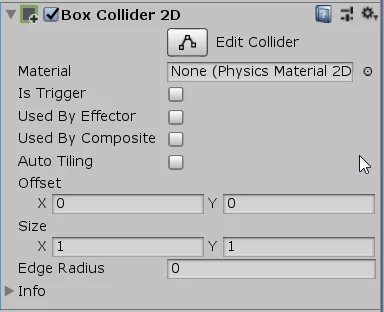Fig. 6: 2-component vectors for the dimensions of a 2D Collider.

In addition to the Unity components, in our Script we can create vectors to use in our algorithms.

The name of the object by which we refer to these vectors in a C# Script for Unity is Vector2 and Vector3. We can see some examples in figure 7.Fig. 7: Examples of the use of mathematical vectors in Scripts C#, in Unity.

## Conclusion

Vectors in mathematics are a useful tool for representing trajectories and forces.

A vector has a module that determines its size, a direction that determines its orientation in space, and a sense within that direction.

Given a vector we can find a single line that contains it.